# 知识蒸馏综述: 知识的类型¶

【GiantPandCV引言】简单总结一篇综述《Knowledge Distillation A Survey》中的内容，提取关键部分以及感兴趣部分进行汇总。这篇是知识蒸馏综述的第一篇，主要内容为知识蒸馏中知识的分类，包括基于响应的知识、基于特征的知识和基于关系的知识。

## 知识蒸馏简介¶

• 知识蒸馏的种类

• 训练机制

• 教师-学生 架构

• 蒸馏算法

• 性能比较

• 实际应用

• 知识 knowledge

• 蒸馏算法 distillation algorithm

• 教师学生架构 teacher-student architecture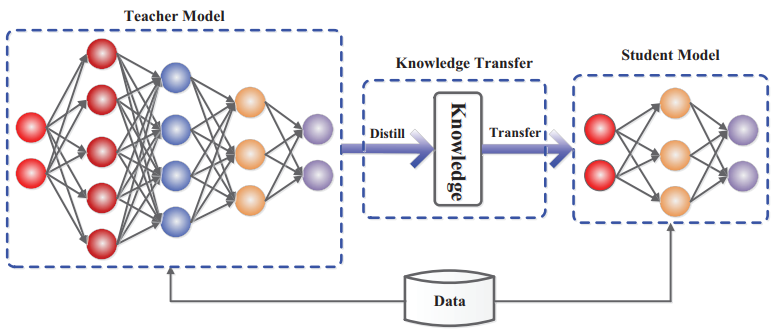• teacher - student learning

• mutual learning

• assistant teaching

• life long learning

• self learning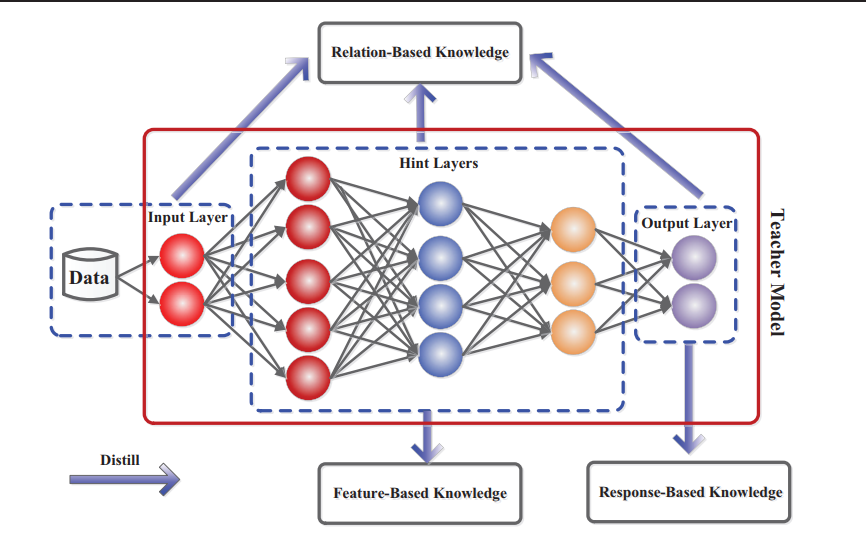## 基于响应的知识（Response-Based Knowledge）¶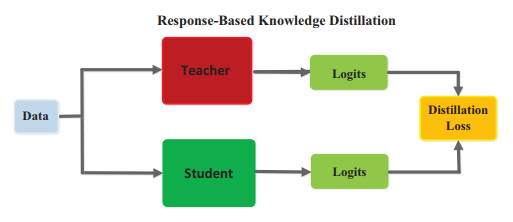L_{R e s D}\left(z_{t}, z_{s}\right)=\mathcal{L}_{R}\left(z_{t}, z_{s}\right)

Hinton提出的KD是将teacher的logits层作为soft label.

p\left(z_{i}, T\right)=\frac{\exp \left(z_{i} / T\right)}{\sum_{j} \exp \left(z_{j} / T\right)}

T是用于控制soft target重要程度的超参数。

L_{\operatorname{Res} D}\left(p\left(z_{t}, T\right), p\left(z_{s}, T\right)\right)=\mathcal{L}_{R}\left(p\left(z_{t}, T\right), p\left(z_{s}, T\right)\right)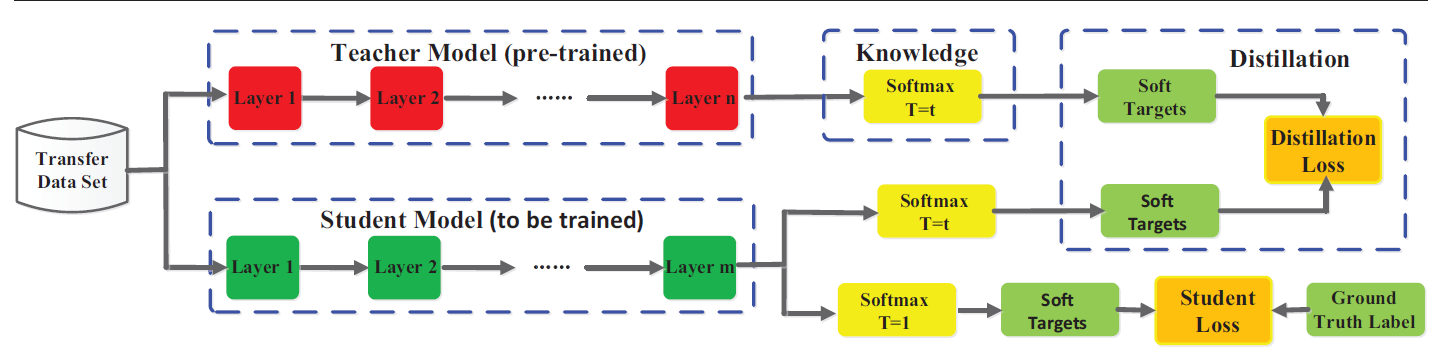KD中除了蒸馏的一项通常还会使用交叉熵损失函数令学生网络学习真实标签（也称hard label)，两项联合起来进行训练。

KD通常的解释是学生网络可以从教师网络中学习到额外的隐形知识（dark knowledge), 而这种知识要比通过标签学习的知识更容易理解。

KD其他角度的解释还包括：KD可以生成类似label smooth的soft target；KD可以视为一种正则化方法；

## 基于特征的知识（Feature-Based Knowledge)¶

FitNets是第一个引入中间层表征的，教师网络的中间层可以作为学生网络对应层的提示（Hints层）从而提升学生网络模型的性能。其核心是期望学生能够直接模仿教师网络的特征激活值。

• Paying more attention to attention: 提出去学习从原先特征图中派生得到的注意力图（Attention map)

• Like what you like: Knowledge distill via neuron selectivity transfer: 认为神经元是有选择性的，而这种选择性与任务是相关的，提出神经元选择性迁移，将教师与学生这种神经元的选择模式分布对齐。通过引入了MMD Matching Loss进行实现。

• Learning deep representation with probabilistic knowledge transfer: 通过匹配特征空间的概率分布迁移知识。

• paraphrasing complex network: Network Compression via factor transfer：引入factor作为一种中间层表征的可理解形式。

• Knowledge distillation via route constrained optimization: 致力于降低教师网络和学生网络的gap,提出使用路线约束的暗示学习(Hints learning)。

• Knowledge transfer via distillation of activation boundaries formed by hidden neurons: 提出使用隐层神经元的激活边界进行知识迁移。

• Cross-Layer Distillation with Sematic Calibration: 为了匹配教师网络和学生网络的语义信息，提出通过attention allocation自适应为学生层分配合适的教师层, 实现跨层的知识蒸馏。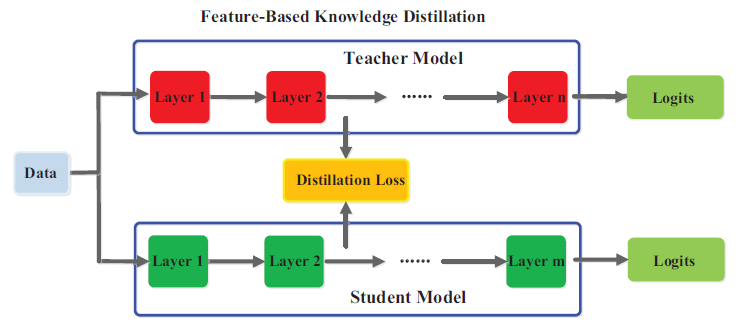L_{F e a D}\left(f_{t}(x), f_{s}(x)\right)=\mathcal{L}_{F}\left(\Phi_{t}\left(f_{t}(x)\right), \Phi_{s}\left(f_{s}(x)\right)\right)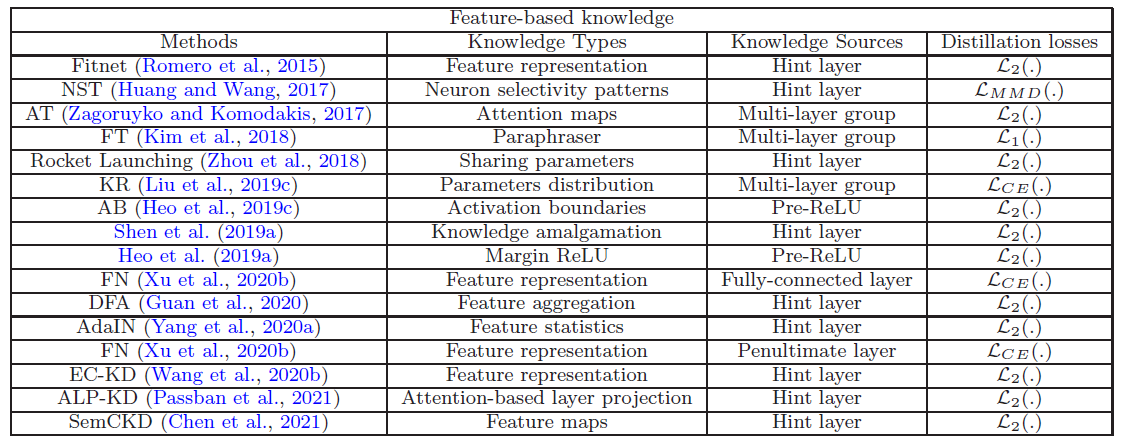• 如何需选择知识类型？特征图、注意力图、gram矩阵或其他

• 如何选择教师层和学生层？简单的一对一匹配，自适应通过注意力匹配。

• 如何弥补教师网络与学生网络之间的GAP？如果容量相差过大，可能会导致学习效果变差。

## 基于关系的知识（Relation-Based Knowledge)¶

### 不同层之间的关系建模¶

• A gift from knowledgedistillation:fast optimization, network minimization and transfer learning: (FSP) 提出了Flow of solution process（FSP）的方法，定义了两个层之间的Gram矩阵，从而可以总结特征图不同对之间的关系。https://github.com/yoshitomo-matsubara/torchdistill/blob/5377be466c9460e0125892aa0d92aeb86418c752/torchdistill/losses/single.py L110行有具体实现。

• self-supervised knowledge distillation using singular value decompostion: 提出利用特征图之间的相关性进行蒸馏，使用奇异值分解的方式来提取特征图中关键信息。

• Better and faster: knowledge transfer from multiple self-supervieds learning tasks via graph distillation for video classification: 为了使用多个教师网络的知识，构建了使用logits层和特征图作为节点的两个graph。

• Graph-based knowledge distillation by multi-head attention network: 提出使用Multi head graph-based 知识蒸馏方法，通过使用graph建模两两feature map之间的关系。

• Heterogeneous Knowledge Distillation using information flow modeling: 提出让学生模仿教师网络信息流动过程来得到知识。

L_{\operatorname{RelD}}\left(f_{t}, f_{s}\right)=\mathcal{L}_{R^{1}}\left(\Psi_{t}\left(\hat{f}_{t}, \check{f}_{t}\right), \Psi_{s}\left(\hat{f}_{s}, \check{f}_{s}\right)\right)

$\Psi_{s}\left(\hat{f}_{s}, \check{f}_{s}\right)$表示学生网络内成对的特征图，$\Psi_{s},\Psi_{t}$代表相似度函数，$\mathcal{L}_{R^{1}}$代表教师网络与学生网络的关联函数。

### 不同样本之间的关系建模¶

• Knowledge distillation via instance relationship graph提出了通过个体及关系图进行知识蒸馏的方法，迁移的知识包括个体级别特征。

• Relational knowledge distillation提出关系知识蒸馏，从个体关系中进行知识迁移

• Learning student networks via feature embedding结合流型学习，学生网络可以通过特征嵌入进行学习，从而保证教师网络中间层特征的样本相似度。

• Probabilistic Knowledge Transfer for Lightweight Deep Representation Learning使用概率分布来建模教师网络和学生网络的关系。

• Similarity-preserving knowledge distillation提出相似性保留的知识，使得教师网络和学生网络根据相同的样本对产生相似的激活。

• Correlation congruence for knowledge distillation提出基于关系一致的知识蒸馏方法，可以同时蒸馏instance-level的信息以及between instance的信息。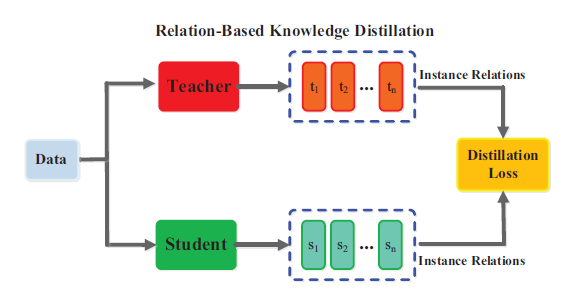instance relation的建模如下：

L_{R e l D}\left(F_{t}, F_{s}\right)=\mathcal{L}_{R^{2}}\left(\psi_{t}\left(t_{i}, t_{j}\right), \psi_{s}\left(s_{i}, s_{j}\right)\right)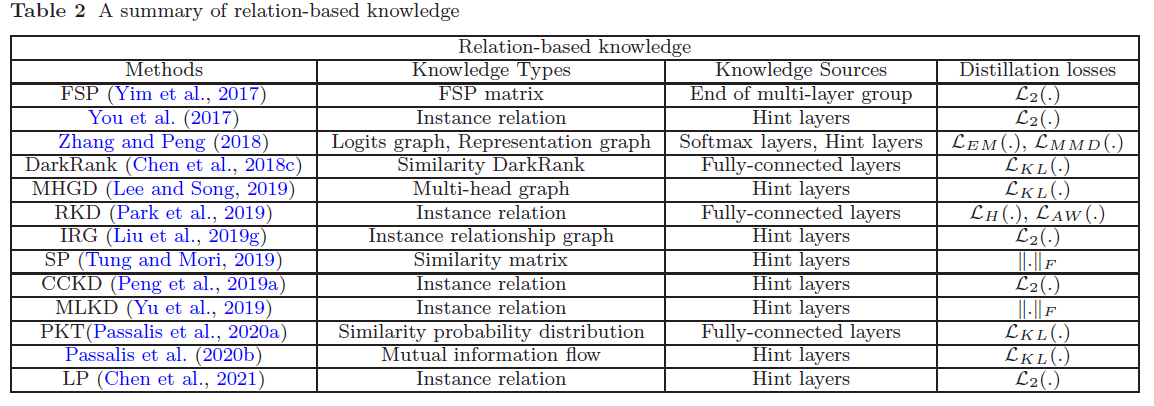## 参考¶

Gou, J., Yu, B., Maybank, S.J., & Tao, D. (2021). Knowledge Distillation: A Survey. ArXiv, abs/2006.05525.

https://arxiv.org/pdf/2006.05525.pdf